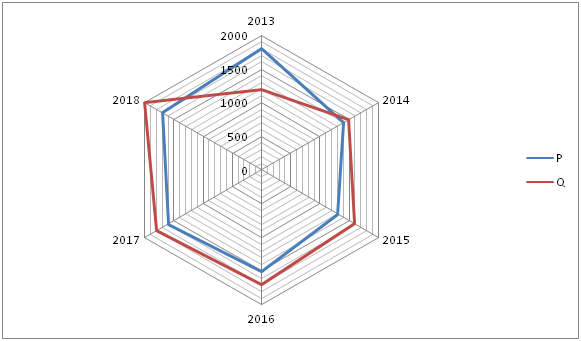# IBPS / LIC Clerk Prelims Quantitative Aptitude Questions 2019 (Day-04)

Dear Aspirants, Our IBPS Guide team is providing new series of Quantitative Aptitude Questions for IBPS Clerk Prelims 2019 so the aspirants can practice it on a daily basis. These questions are framed by our skilled experts after understanding your needs thoroughly. Aspirants can practice these new series questions daily to familiarize with the exact exam pattern and make your preparation effective.

Check here for IBPS Clerk Prelims Mock Test 2019

##### Check here for LIC Assistant Prelims Mock Test 2019
[WpProQuiz 7240]

Missing Number Series

Directions (Q. 1 – 5): What value should come in place of (?) in the following number series?

1) 250, 126, 64, 33, ?

a) 16

b) 13.5

c) 17.5

d) 18

e) 22

2) 132, 132, 134, 140, 152, ?

a) 172

b) 216

c) 188

d) 234

e) 196

3) 56, 27, 26, 38, 75, ?

a) 276

b) 248

c) 162

d) 186.5

e) 206.5

4) 158, 170, 186, 210, 246, ?

a) 312

b) 298

c) 336

d) 384

e) 372

5) 156, 78, 52, 39, ? , 26

a) 33

b) 29

c) 31.2

d) 32.4

e) 34

Data Interpretation

Directions (Q. 6 – 10): Study the following information carefully and answer the given questions?

The following radar graph shows the total number of students studied in two different schools in 6 different years.6) Find the difference between the total number of students studied in School P in the year 2013 and 2015 together to that of total number of students studied in School Q in the year 2014 and 2017 together?

a) 200

b) 250

c) 150

d) 300

e) None of these

7) Find the average number of students studied in School P in all the given years together?

a) 1700

b) 1400

c) 1250

d) 1350

e) None of these

8) Find the ratio between the total numbers of students studied in School Q in 2013 to that of total number of students studied in School P in 2016?

a) 1: 3

b) 5: 7

c) 4: 5

d) 9: 11

e) None of these

9) Total number of students studied in School Q in the year 2015 and 2016 together is approximately what percentage of total number of students studied in School P in the year 2014 and 2018 together?

a) 115 %

b) 144 %

c) 162 %

d) 106 %

e) 128 %

10) Find the difference between the average number of students studied in School P in the year 2013, 2015 and 2018 together to that of the average number of students studied in School Q in the year 2013, 2014 and 2017 together?

a) 200

b) 300

c) 250

d) 100

e) None of these

Directions (1-5) :

250 ÷ 2 + 1 = 126

126 ÷ 2 + 1 = 64

64 ÷ 2 + 1 = 33

33 ÷ 2 + 1 = 17.5

132 + (12 – 1) = 132

132 + (22 – 2) = 134

134 + (32 – 3) = 140

140 + (42 – 4) = 152

152 + (52 – 5) = 172

56 * 0.5 – 1 = 27

27 * 1 – 1 = 26

26 * 1.5 – 1 = 38

38 * 2 – 1 = 75

75 * 2.5 – 1 = 186.5

158,            170,            186,            210,            246,            298

12               16               24               36               52

4                 8                 12               16

The difference of difference is, 4, 8, 12, 16,…

156 * (1/2) = 78

78 * (2/3) = 52

52 * (3/4) = 39

39 * (4/5) = 31.2

31.2 * (5/6) = 26

Directions (6-10) :

The total number of students studied in School P in the year 2013 and 2015 together

= > 1800 + 1300 = 3100

The total number of students studied in School Q in the year 2014 and 2017 together

= > 1500 + 1800 = 3300

Required difference = 3300 – 3100 = 200

The average number of students studied in School P in all the given years together

= > [1800 + 1400 + 1300 + 1500 + 1600 + 1700]/6

= > [9300/6] = 1550

The total number of students studied in School Q in 2013 = 1200

The total number of students studied in School P in 2016 = 1500

Required ratio = 1200: 1500 = 4: 5

Total number of students studied in School Q in the year 2015 and 2016 together

= > 1600 + 1700 = 3300

Total number of students studied in School P in the year 2014 and 2018 together

= > 1400 + 1700 = 3100

Required % = (3300/3100) * 100 = 106.45 % = 106 %

The average number of students studied in School P in the year 2013, 2015 and 2018 together

= > (1800 + 1300 + 1700)/3 = 1600

The average number of students studied in School Q in the year 2013, 2014 and 2017 together

= > (1200 + 1500 + 1800)/3 = 1500

Required difference = 1600 – 1500 = 100